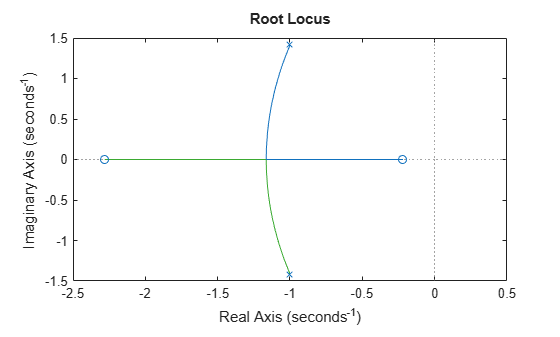Documentation

# rlocus

Root locus plot of dynamic system

## Syntax

```rlocus(sys) rlocus(sys1,sys2,...) [r,k] = rlocus(sys) r = rlocus(sys,k) ```

## Description

`rlocus ` computes the root locus of a SISO open-loop model. The root locus gives the closed-loop pole trajectories as a function of the feedback gain k (assuming negative feedback). Root loci are used to study the effects of varying feedback gains on closed-loop pole locations. In turn, these locations provide indirect information on the time and frequency responses.

`rlocus(sys)` calculates and plots the root locus of the open-loop SISO model `sys`. This function can be applied to any of the following negative feedback loops by setting `sys` appropriately.If `sys` has transfer function

`$h\left(s\right)=\frac{n\left(s\right)}{d\left(s\right)}$`

the closed-loop poles are the roots of

`$d\left(s\right)+kn\left(s\right)=0$`

`rlocus` adaptively selects a set of positive gains k to produce a smooth plot. Alternatively,

```rlocus(sys,k) ```

uses the user-specified vector `k` of gains to plot the root locus.

`rlocus(sys1,sys2,...)` draws the root loci of multiple LTI models` sys1, sys2,...` on a single plot. You can specify a color, line style, and marker for each model, as in

```rlocus(sys1,'r',sys2,'y:',sys3,'gx'). ```

`[r,k] = rlocus(sys)` and `r = rlocus(sys,k)` return the vector `k` of selected gains and the complex root locations `r` for these gains. The matrix `r` has `length(k)` columns and its `j`th column lists the closed-loop roots for the gain `k(j)`.

## Examples

collapse all

Plot the root-locus of the following system.

`$h\left(s\right)=\frac{2{s}^{2}+5s+1}{{s}^{2}+2s+3}.$`

```h = tf([2 5 1],[1 2 3]); rlocus(h)```You can use the right-click menu for rlocus to add grid lines, zoom in or out, and invoke the Property Editor to customize the plot. Also, click anywhere on the curve to activate a data marker that displays the gain value, pole, damping, overshoot, and frequency at the selected point.

## Tips

You can change the properties of your plot, for example the units. For information on the ways to change properties of your plots, see Ways to Customize Plots.